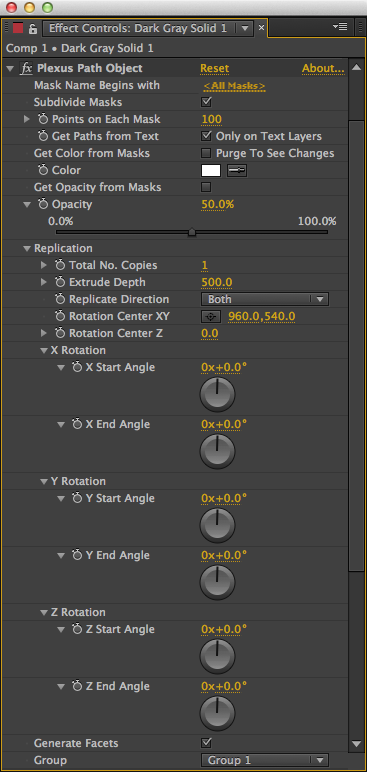## Paths ObjectMask Name Begins With: Only Masks that begin with this name will considered by the effect. All the other masks in the applied to the layer are ignored.

Subdivide Masks: Subdivides the Masks into additional number of points. If unselected, only the original vertices are considered.

Points on Each Mask: Number of Additional Points on Each Mask, if Subdivide masks is selected.

Get Paths From Text: If Selected, the Path outlines from text layers are considered.

Get Color from Masks: Get the Color from the Mask Color. To see changes, make sure to purge since AE doesn’t report changes to the color of the Mask actively to the effect. This overrides the default color of the points.

Get Opacity From Masks: Get the Opacity from Mask Opacity. This overrides the default opacity of the points.

Color: The default color of the points.

Opacity: The default opacity of the points.

Replication: The points created by this object can replicated in 3D space.

Total No. Copies: The number of Objects to be created. By default only one instance is created.

Extrude Depth: If multiple copies are created, they are extruded to a certain depth.

Replicate Direction: The direction of the extrusion. Can be Positive Z axis/Negative Z Axis/ Both.

Rotation Center XY: The XY coordinates of the Rotation center, by which all the copies are replicated.

Rotation Center Z: The Z coordinate of the Rotation Center, by which all the copies are replicated.

X Start Angle: The X Rotation Angle of the first instance.

X End Angle: The X Rotation Angle of the Last instance.

All the X Rotation angles of the instances in between are calculated based on the X Start Angle and the X End Angle.

Y Start Angle: The Y Rotation Angle of the first instance.

Y End Angle: The Y Rotation Angle of the Last instance.

All the Y Rotation angles of the instances in between are calculated based on the Y Start Angle and the Y End Angle.

Z Start Angle: The Z Rotation Angle of the first instance.

Z End Angle: The Z Rotation Angle of the Last instance.

All the Z Rotation angles of the instances in between are calculated based on the Z Start Angle and the Z End Angle.

Generate Faces: Generate Faces between the vertices, which can be rendered using the Facets Render Object.

Group: The Group which the Object belongs to in the Plexus.